Wind Energ. Sci., 6, 1031–1041, 2021
https://doi.org/10.5194/wes-6-1031-2021
Wind Energ. Sci., 6, 1031–1041, 2021
https://doi.org/10.5194/wes-6-1031-2021

Research article 29 Jul 2021

Research article | 29 Jul 2021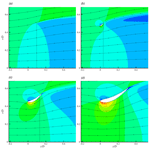# Maximal power per device area of a ducted turbine

Maximal power per device area of a ducted turbine
Nojan Bagheri-Sadeghi, Brian T. Helenbrook, and Kenneth D. Visser Nojan Bagheri-Sadeghi et al.
• Department of Mechanical and Aeronautical Engineering, Clarkson University, Potsdam, NY 13699-5725, United States

Abstract

The aerodynamic design of a ducted wind turbine for maximum total power coefficient was studied numerically using the axisymmetric Reynolds-averaged Navier–Stokes equations and an actuator disc model. The total power coefficient characterizes the rotor power per total device area rather than the rotor area. This is a useful metric to compare the performance of a ducted wind turbine with an open rotor and can be an important design objective in certain applications. The design variables included the duct length, the rotor thrust coefficient, the angle of attack of the duct cross section, the rotor gap, and the axial location of the rotor. The results indicated that there exists an upper limit for the total power coefficient of ducted wind turbines. Using an Eppler E423 airfoil as the duct cross section, an optimal total power coefficient of 0.70 was achieved at a duct length of about 15 % of the rotor diameter. The optimal thrust coefficient was approximately 0.9, independent of the duct length and in agreement with the axial momentum analysis. Similarly independent of duct length, the optimal normal rotor gap was found to be approximately the duct boundary layer thickness at the rotor. The optimal axial position of the rotor was near the rear of the duct but moved upstream with increasing duct length, while the optimal angle of attack of the duct cross section decreased.

Share
1 Introduction

The power output of a wind turbine can be augmented by surrounding it with a duct, typically referred to as a ducted wind turbine (DWT), a diffuser augmented wind turbine, or a shrouded wind turbine. The effect of the duct is to increase the mass flow rate through the rotor. For a given rotor area, significantly more power can be obtained for a DWT compared to an open wind turbine. However, by adding a duct, the total area of the device facing the wind direction is increased. If the power produced per total projected frontal area of the device is calculated for DWTs, often values closer to that of open wind turbines are found . When nondimensionalized by the kinetic power available in a unit area of freestream, the power per rotor area and power per total area of the DWT are referred to as rotor and total power coefficients and are denoted by CP and CP,total respectively. For an open rotor turbine, these two power coefficients are equal. Achieving values of CP,total greater than the Betz–Joukowsky limit of 0.593 for a DWT is significant as it means a DWT can capture more power per unit area of the device than an open rotor turbine. Optimization studies of DWTs have shown that DWTs can achieve values of CP,total beyond the Betz–Joukowsky limit . CP,total not only is a useful metric to compare DWTs with open rotor wind turbines but could be an important design objective in certain problems like fully integrated DWTs for sustainable buildings or other applications where a designer seeks to maximize the power output from the limited space allocated to a wind turbine.

Since the experimental demonstration of the power augmentation provided by shrouding wind turbines (Sanuki1950), numerous studies on the design and optimization of the DWTs have been carried out . Only a few have used CP,total as a design metric, while most other studies have focused on maximizing CP. The design variables of a DWT include the rotor blade design, its axial location (zrotor), tip clearance or rotor gap (Δn), the angle of attack (α), length (l), and shape of the duct cross section. These design variables can be seen in the schematic shown in Fig. 1 with the rotor replaced by an actuator disc.

Many of the numerical studies use axisymmetric computational fluid dynamics (CFD) models . If the rotor blades are modeled as an actuator disc, the thrust coefficient can be considered a design variable, and different rotor loadings can be represented by different values of the thrust coefficient . Similarly, some experimental studies replace turbines with screens of different porosity to study DWTs at various rotor loadings . Most simplified theoretical models of DWTs indicate that the optimal power output is achieved at a thrust coefficient of $\mathrm{8}/\mathrm{9}$ similar to open wind turbines . reviewed various theoretical models of ducted wind turbines and concluded that they are all equivalent and that their apparent differences are due to different choices of flow parameters used to characterize the effect of the duct (e.g., the exit pressure coefficient, ; or extra back pressure velocity ratio, ). They also show that these models are insufficient in predicting the optimal design of ducted turbines as they neglect the dependence of the flow parameters on the thrust coefficient. However, they demonstrated that CP,total greater than the Betz–Joukowsky limit can be achieved at ${C}_{\mathrm{T}}=\mathrm{8}/\mathrm{9}$. reported an optimal value of thrust coefficient close to this value when optimizing for CP,total using Reynolds-averaged Navier–Stokes (RANS) simulations with an actuator disc model. However, performed axisymmetric inviscid simulations of DWTs with various duct shapes with an actuator disc and concluded that the value of CT to maximize CP is lower than $\mathrm{8}/\mathrm{9}$.

As most studies focus on maximizing CP, with a few exceptions , the axial location of the rotor is usually fixed at the smallest cross section of the duct where the maximum velocity is assumed. In our previous paper , we included the axial position of the rotor as a design variable and compared the optimal designs for maximum CP and CP,total. We observed that the optimal axial location of a rotor to maximize CP or power is close to the duct throat. However, when optimizing for maximum CP,total, the optimal axial position moves further downstream of the duct throat, which for a given rotor size results in a significantly smaller total area of the device.

The effect of the angle of attack of the duct cross section has been included in most studies . The results of these studies show that power output is considerably sensitive to the angle of attack of the duct cross section and that more power is obtained by increasing the angle of attack of the duct cross section up to the point where the flow separates inside the duct. As noted by , the optimal design of a DWT is on the verge of flow separation, which is often accompanied by a sharp decrease in the power output. Therefore, the accuracy of CFD simulations significantly depends on the accurate prediction of flow separation. The kω shear-stress transport (SST) turbulence model (Menter1994) is more accurate than the kϵ models in prediction of the flow separation for flows with adverse pressure gradients and thus is preferred when RANS simulations are used to study DWTs . This sharp drop in the power, which results in a discontinuity in the objective function, has implications in the choice of optimization method too, as it renders methods assuming a smooth objective function ineffective .

There are a few studies on the optimization of the shape of the duct cross section for optimal CP,total such as , but the design space is limited by fixing some other design variables. For instance, use a penalty function to avoid large values of the thrust coefficient, and the axial location of the rotor, the chord length of the duct cross section, and the rotor gap were not introduced as design variables. In most studies, a high-lift airfoil with the suction side inside the duct is used as the cross section of the duct (de Vries1979). A high-lift airfoil shape creates circulation and thereby increases the mass flow rate through the duct. Further increases in CP,total could be possible by delaying boundary layer separation, e.g., by using multi-element or slotted ducts , as the optimal design tends to be on the verge of flow separation . Large flanges are often used at the exit of ducted turbines to further lower the pressure at the exit plane of the duct and increase the swallowing effect. showed that large flanges lead to reduced values of CP,total.

The effect of the rotor gap as a design variable is considered in a few studies , and although optimum rotor gaps have been examined , no conclusions about the optimal rotor gap for optimal CP,total have been obtained to our knowledge. Lastly, the effect of the duct length for a given rotor diameter on the optimal design of a DWT is only considered in a few works . Within the range of duct lengths that seem practical (lengths smaller than the rotor diameter), studies suggest that CP can be increased monotonically by increasing the duct length. However, we are not aware of any studies on the effect of the duct length on the optimal design for CP,total.

This study investigates the effect of the duct length on the optimal design for maximizing the total power coefficient (CP,total) of a DWT having the Eppler E423 airfoil as the cross section. This entails identifying whether there is an optimal duct length and how the optimal design variables of a DWT change as the duct length varies. The results show that there is an upper limit to CP,total for a DWT, which is similar to the Betz–Joukowsky limit for open rotors. The result established here is specific to the Eppler E423 used for the duct cross section, but a similar result should hold for other duct cross sections as well. Additionally, the results indicate that the optimal rotor gap is close to the boundary layer thickness at the rotor independent of the duct length. Furthermore, this paper illustrates how the optimal axial position and the angle of attack of the duct change with increasing duct length.

The paper is organized as follows: Sect. 2 discusses the CFD model used for RANS simulations including a validation study of the actuator disc model used followed by the details of the DWT design parameterization and the optimization method used. The optimization results and how the optimal design changes with the duct length are discussed in the third section. This section also involves a sensitivity analysis of CP,total of the optimal design, as well as a comparison of the power per unit device area vs. rotor thrust of the optimal DWT and an open rotor.

2 Methods

Ansys Fluent 17.1 was used to solve the incompressible RANS equations with the kω SST turbulence model (Menter1994). The computational domain used is shown in Fig. 2, which extends to max(4D,15c) upstream of the rotor and max(8D,25c) downstream of it, where max(x,y) is the greater of x and y, D is the rotor diameter, and c is the chord length of the duct cross section. The flow was considered axisymmetric, and the rotor was modeled as an actuator disc. The pressure drop across each cell of the actuator disc was

$\begin{array}{}\text{(1)}& \mathrm{\Delta }p=\frac{\mathrm{1}}{\mathrm{2}}\mathit{\rho }{V}_{z}^{\mathrm{2}}{C}_{\mathrm{T},\mathrm{rotor}},\end{array}$

where ρ is the air density and CT,rotor is the thrust coefficient based on the axial velocity (Vz) at the rotor. The value of CT,rotor was defined in the fan boundary condition of Ansys Fluent. Using CT,rotor as a design variable means that the pressure drop across the actuator disc was not constant. However, as it is easier to interpret, all the results are given in terms of the thrust coefficient defined as ${C}_{\mathrm{T}}=\frac{T}{\frac{\mathrm{1}}{\mathrm{2}}\mathit{\rho }{V}_{\mathrm{\infty }}^{\mathrm{2}}{A}_{\mathrm{rotor}}}$, where T is the thrust force on the rotor, V is the freestream velocity, and Arotor is the swept area of the rotor. At the inlet, the freestream values of the turbulence variables were set as ${\mathit{\omega }}_{\mathrm{\infty }}=\frac{\mathrm{5}{V}_{\mathrm{\infty }}}{D}$ and ${k}_{\mathrm{\infty }}=\mathit{\nu }{\mathit{\omega }}_{\mathrm{\infty }}×{\mathrm{10}}^{-\mathrm{3}}$, where ν is the kinematic viscosity of air. The flow field of RANS actuator disc simulations are sensitive to freestream values of turbulence variables , and the values selected correspond to recommended values by . Ansys Fluent uses a cell-centered finite-volume method. The pressure-based solver with the coupled algorithm and Fluent's second-order accurate schemes were used for all flow variables. The values of rotor power output and thrust were monitored to ensure iterative convergence.

The grid near the duct, which uses both structured and unstructured elements, is depicted in Fig. 3. The average nondimensional wall distance of the first grid point in the boundary layer mesh was ${y}_{\mathrm{1}}^{+}\approx \mathrm{1}$, and the aspect ratio of the first element on the airfoil was set to about 20. The thickness of the boundary layer mesh was set equal to $\mathrm{min}\left(\mathit{\delta },\mathrm{0.95}\mathrm{\Delta }n/c\right)$, where δ is the thickness of the boundary layer over the airfoil estimated from the flat-plate correlation $\mathit{\delta }=\mathrm{1.1}\frac{\mathrm{0.16}c}{R{e}_{\mathrm{c}}^{\mathrm{1}/\mathrm{7}}}$, where the 1.1 factor accounts for the longer curved surface of the airfoil compared to its chord (White2006). This prevents the actuator disc from penetrating the boundary layer mesh, which can result in the failure of boundary layer mesh generation. The fan boundary condition in Fluent requires identifying the direction of the positive pressure jump. The use of triangular elements as shown in Fig. 3 between the boundary layer mesh and the outside structured mesh made the grid generation more efficient. However, with the triangular elements used on the fan boundary condition, the specified direction of the fan boundary condition randomly changed from case to case, and sometimes the actuator disc became undesirably distorted. To fix this issue, a thin structured quadrilateral grid was created just downstream of the fan boundary, which is seen in Fig. 3. The width and cell sizing of this thin grid are scaled with $c/D$ to prevent large cells near the boundary layer mesh for smaller ducts.

Two metrics were used to characterize the performance of a DWT: first, the power coefficient based on the swept area of the rotor,

$\begin{array}{}\text{(2)}& {C}_{\mathrm{P}}=\frac{P}{\frac{\mathrm{1}}{\mathrm{2}}\mathit{\rho }{V}_{\mathrm{\infty }}^{\mathrm{3}}{A}_{\mathrm{rotor}}},\end{array}$

and, second, the power coefficient based on the exit area of the duct,

$\begin{array}{}\text{(3)}& {C}_{\mathrm{P},\mathrm{total}}=\frac{P}{\frac{\mathrm{1}}{\mathrm{2}}\mathit{\rho }{V}_{\mathrm{\infty }}^{\mathrm{3}}{A}_{\mathrm{total}}}.\end{array}$

CP,total is a measure of the performance for a given total cross-sectional area of the device, whereas CP is the performance for a given rotor cross-sectional area.

In order to validate the actuator disc model, the axisymmetric actuator disc without a duct was simulated in the domain shown in Fig. 2. Figure 4 compares the axisymmetric RANS actuator disc simulation results on three different grids with the 1-D actuator disc momentum theory. The coarse, medium, and fine grids had about 1.6×104, 6.5×104, and 2.6×105 cells. The only noticeable difference between the three grids is observed at values of CT close to 1. At lower values of CT the RANS actuator disc model and the momentum theory results are visually indistinguishable. For heavily loaded actuator discs with CT>1, the simple 1-D momentum theory is not valid and empirical correlations are often used .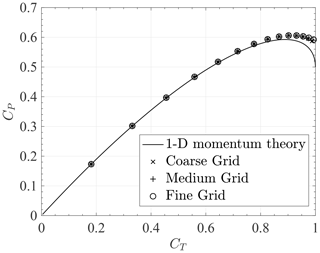Figure 4Comparison of axisymmetric RANS actuator disc model with 1-D momentum actuator disc theory.

## 2.1 Optimization

The performance of the DWT was considered to be a function of a number of design variables, mentioned in the introduction, including the chord length of the duct cross section (c), the thrust coefficient based on the axial velocity at the rotor (CT,rotor), the angle of attack of the duct cross section (α), the normal rotor gap (Δn), and the axial position of the rotor (zrotor). These are shown in Fig. 1. The optimization problem was to maximize CP,total as a function of normalized design variables $\frac{c}{D}$, CT,rotor, α, $\frac{\mathrm{\Delta }n}{c}$, and $\frac{{z}_{\mathrm{rotor}}}{l}$, where l is the duct length as shown in Fig. 1. Although the design variable was CT,rotor, all the results are presented in terms of the easier-to-interpret thrust coefficient based on the freestream velocity ${C}_{\mathrm{T}}=\frac{T}{\frac{\mathrm{1}}{\mathrm{2}}\mathit{\rho }{V}_{\mathrm{\infty }}^{\mathrm{2}}{A}_{\mathrm{rotor}}}$, where $T=\underset{\mathrm{0}}{\overset{D/\mathrm{2}}{\int }}\mathrm{2}\mathit{\pi }\mathrm{\Delta }pr\mathrm{d}r$. The constraints of the optimization were the positivity of $c/D$, CT, α, and $\mathrm{\Delta }n/c$ and $\mathrm{0}<{z}_{\mathrm{rotor}}/l<\mathrm{1}$. The nondimensionalization of the rotor gap by chord length instead of rotor diameter was done to help the optimization process as the optimal normal rotor gap seemed to scale with the chord length of the duct cross section in general. The results given in the next section support this scaling.

When $c/D$ is included as a design variable, one can fix the value of Rec or ReD. If ReD is fixed, the smaller values of $c/D$ result in values of Rec lower than the operating range of the airfoil for which Reynolds number dependency can be expected. Additionally, the larger values of $c/D$ result in high values of Rec, which require more computationally expensive boundary layer meshes. For this reason, Rec was fixed and ReD was allowed to vary. Rec was set to a value of 3×105. For the Eppler E423 airfoil used here, the operating range extends to $R{e}_{\mathrm{c}}=\frac{{V}_{\mathrm{\infty }}c}{\mathit{\nu }}$ as low as 1.4×105. For lower Reynolds numbers, large flow separation is observed before the airfoil can attain its design maximum lift coefficient . For the range of chord lengths studied, ReD varied from 6.0×105 to 6.0×106. The axisymmetric actuator disc model used here is insensitive to the Reynolds number once ReD is greater than 2000 . Within this range of ReD, the value of CP only changed by about 0.07 % when using the fine grid of the actuator disc validation study at ${C}_{\mathrm{T}}=\mathrm{8}/\mathrm{9}$. Therefore, fixing Rec should minimize any Reynolds number effects and keep the computational expense of cases with larger $c/D$ manageable. To determine Reynolds number sensitivity, the optimization was repeated at $R{e}_{\mathrm{c}}=\mathrm{1.2}×{\mathrm{10}}^{\mathrm{6}}$ using the optimal design at $R{e}_{\mathrm{c}}=\mathrm{3}×{\mathrm{10}}^{\mathrm{5}}$ as the starting point.

The Hooke and Jeeves direct search optimization technique was employed in this study. Our optimization study concluded that the flow inside the duct of an optimal design for either CP or CP,total as the objective function is on the verge of separation. The flow separation inside the duct can result in a significant drop in power output, and therefore the objective function can be considered almost discontinuous close to such optimal design points. The performance of optimization methods that rely on objective function gradients is affected by the presence of such a discontinuity. The Hooke and Jeeves method, however, is less affected by such discontinuities as it does not assume a smooth objective function and only uses the objective function values to identify if a better design point is found or not.

The optimization technique starts by modifying the initial design, one design variable at a time. These exploratory moves in the design space are called steps in coordinate directions. All the design variables were scaled by their initial values, and the initial step size in each coordinate direction was set to 5 %. Based on the success or failure of these steps in the coordinate directions of the five-dimensional design space, the algorithm creates pattern directions, moves the base point, and increases or decreases the step sizes. The stopping criterion was $\sum _{i=\mathrm{1}}^{N}\frac{\mathrm{1}}{N}|\frac{\mathrm{\Delta }{x}_{i}}{\mathrm{\Delta }{x}_{i,\mathrm{0}}}|<\mathrm{0.1}$, where Δxi is the step size in each coordinate direction, Δxi,0 is the initial step size, and N is the number of design variables. This means that the initially small step sizes should on average reduce by an order of magnitude for the optimization to stop. Figure 5 shows the convergence of the optimization technique towards the optimal design. The optimization was repeated with a different initial design, and the optimization approached the same optimal design point.

Table 1The design variables for optimal CP,total.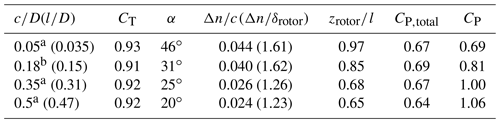a The optimization performed at fixed $c/D$ at $R{e}_{\mathrm{c}}=\mathrm{3}×{\mathrm{10}}^{\mathrm{5}}$.
b The optimization performed with $c/D$ as a design variable at $R{e}_{\mathrm{c}}=\mathrm{3}×{\mathrm{10}}^{\mathrm{5}}$.

3 Results and discussion

The optimization was performed at a constant $R{e}_{\mathrm{c}}=\mathrm{3}×{\mathrm{10}}^{\mathrm{5}}$ as described in the Methods section. The optimal design achieved ${C}_{\mathrm{P},\mathrm{total}}=\mathrm{0.69}$ at $c/D=\mathrm{0.18}$, which corresponds to $l/D=\mathrm{0.15}$. The values of design variables and duct length at this optimal design are given in the second row of Table 1. The optimal design was also simulated on a finer grid with about 1.11×106 cells compared to 2.8×105 cells of the original grid. There was only a 0.03 % difference between CP,total values. Also, simulating on a larger domain extending 1.4 times further in each direction, the value of CP,total changed by about −1 %, which was considered sufficiently accurate for the optimization study carried out here.

To verify that there is indeed a maximum in CP,total with duct length, optimizations were carried out at several other fixed $c/D$ values at $R{e}_{\mathrm{c}}=\mathrm{3}×{\mathrm{10}}^{\mathrm{5}}$. The optimal designs at these fixed values of $c/D$ are shown in Table 1 as well. Figure 6 shows the power coefficients of the designs for optimal CP,total. These additional optimization studies confirm that there is an optimal duct length for a DWT that maximizes CP,total and that this maximum is greater than the Betz–Joukowsky limit. Thus, for applications desiring the greatest power per unit device area, the duct length should be around 15 % of the rotor diameter. As the results here are specific to Eppler E423, further studies are needed to verify this conclusion. The values of power coefficient based on the rotor swept area (CP) are also shown in Fig. 6 for the designs of Table 1. The values of CP seem to increase almost linearly with $c/D$. This suggests that significantly larger values of CP can be obtained by increasing the duct length, but this will result in a lower CP,total.

Both here and in the values of optimal CT were close to 0.9. For all optimal designs of Table 1, the value of the thrust coefficient is close to ${C}_{\mathrm{T}}=\mathrm{8}/\mathrm{9}$, which is the optimal thrust coefficient of open wind turbines at the Betz–Joukowsky limit. This is in agreement with the momentum analysis and CFD studies of DWTs, which conclude that the optimal CT values of open and ducted wind turbines are similar .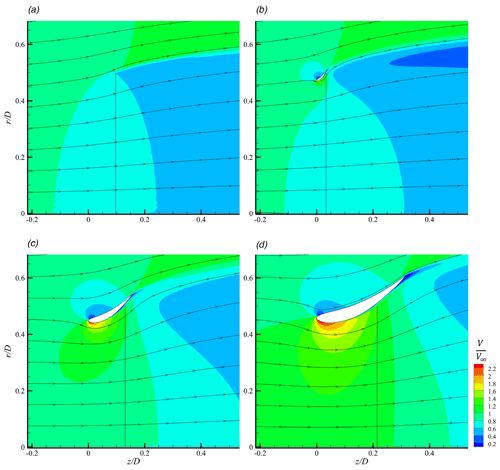Figure 7The streamlines and contours of nondimensional velocity magnitude $V/{V}_{\mathrm{\infty }}$ of (a) the actuator disc, shown by the radial black line, at ${C}_{\mathrm{T}}=\mathrm{8}/\mathrm{9}$, and the designs for optimal CP,total at $R{e}_{\mathrm{c}}=\mathrm{3}×{\mathrm{10}}^{\mathrm{5}}$ at (b) $c/D=\mathrm{0.05}$, (c) $c/D=\mathrm{0.18}$, and (d) $c/D=\mathrm{0.35}$.

The geometry and streamlines of the first three configurations in Table 1 are shown in Fig. 7b–d along with those for an open rotor (in Fig. 7a). The optimal angle of attack of the duct cross section decreased with increasing duct length. For the open rotor, the streamlines close to the tip are at an angle of about 30. Furthermore, at $c/D=\mathrm{0.05}$ almost all of the duct cross section can be considered to be in the vicinity of the strong divergence of streamlines close to the rotor tip. This explains why for the small $c/D=\mathrm{0.05}$ the flow is still attached at α=46. The presence of the small duct with ${z}_{\mathrm{rotor}}/l=\mathrm{0.98}$ adds extra suction inside the duct without significantly increasing the total area of the device and achieves about 10 % more power per unit device area than an open rotor (i.e., 10 % more than the optimal power output of an actuator disc RANS simulation, which was about 0.6 as shown in Fig. 4). For the optimal design with a variable $c/D$ (i.e., $c/D=\mathrm{0.18}$), the increase in CP,total is 15 % over an open rotor, and the angle of attack is reduced to 31. Note that the angle of streamlines of the actuator disc with respect to the horizontal decreases further downstream as the rotor wake recovers in Fig. 7. Similarly, further upstream of the rotor for the actuator disc case, the angle of streamlines decreases. At greater duct lengths, the airfoil of the duct cross section becomes less influenced by the presence of the actuator disc and hence the maximum α without a large flow separation decreases. This trend continues for $c/D=\mathrm{0.5}$ (see Table 1).

The optimal normal rotor gap ($\mathrm{\Delta }n/c$) decreased with increasing duct length, but the ratio of the rotor gap to the estimated boundary layer thickness at the rotor ($\mathrm{\Delta }n/{\mathit{\delta }}_{\mathrm{rotor}}$) stayed nearly constant. A smaller rotor gap means a smaller exit area of the duct, and therefore it helps to increase CP,total. On the other hand, the presence of the rotor gap results in an annular jet of high-velocity air which imparts momentum to the boundary layer and helps the flow stay attached. A Δn that is too small or too large weakens this annular jet. The jet is easier to see in the contour plot of $c/D=\mathrm{0.35}$ in Fig. 7d. For all of the optimal cases shown in Table 1, $\mathrm{\Delta }n/{\mathit{\delta }}_{\mathrm{rotor}}$ was close to 1. When the chord is small relative to D, the dominant length scale is c. This determines the flow scales as well as the boundary layer thickness. Also, note that because Rec is held constant, the boundary layer thickness at the trailing edge δ scales linearly with c. Therefore, as optimal $\mathrm{\Delta }n/{\mathit{\delta }}_{\mathrm{rotor}}$ is nearly constant, optimal $\mathrm{\Delta }n/c$ only slightly decreases as the optimal rotor position is moved further upstream, resulting in a smaller boundary layer thickness at the rotor (δrotor) compared to the trailing edge. This justifies the scaling of Δn by the chord length of the duct.

Similar to , the design for optimal CP,total resulted in downstream placement of the rotor. The optimal rotor position moved further upstream in the duct as $c/D$ increased. Note that at greater duct lengths the annular jet is stronger as can be seen in Fig. 7. This further upstream placement of the rotor means that the annular jet formed between the rotor tip and duct can exchange momentum with a larger portion of the boundary layer, which may help the DWT attain more power per total unit device area without flow separation.

The sensitivity of the total power coefficient of the optimal design to different design variables xi is shown in Fig. 8. The greatest sensitivity is to the thrust coefficient of the rotor which matches the results of previous studies and illustrates the importance of rotor design in achieving optimal power output from a DWT (a rotor design approach based on the blade element momentum method using axisymmetric RANS actuator disc simulations as input is discussed by ). For α and ${z}_{\mathrm{rotor}}/l$, an increase in the design variable causes flow separation inside the duct and a significant drop in the power output. This is demonstrated by an increased sensitivity of CP,total to increase in α and ${z}_{\mathrm{rotor}}/l$ compared to their reduction. The sensitivity to the reduction in ${z}_{\mathrm{rotor}}/l$ is mainly driven by changes in the total area of the device rather than changes in the power output. Therefore as concluded in a smaller DWT with similar power output (i.e., a greater CP,total) can be designed by placing the rotor further downstream of the duct. The observation that the optimal design is on the verge of flow separation with respect to α agrees with the results of obtained for the design for optimal CP. The flow of the design for optimal CP in is on the verge of separation with respect to rotor gap and shows little sensitivity to ${z}_{\mathrm{rotor}}/l$, whereas the optimal design for CP,total shows the least sensitivity in Fig. 8 to $\mathrm{\Delta }n/c$, and a slight increase in ${z}_{\mathrm{rotor}}/l$ leads to flow separation.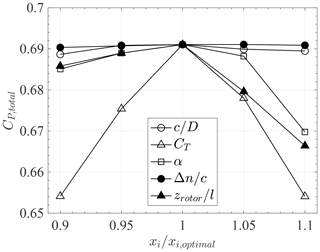Figure 8The sensitivity of the total power coefficient of the optimal design to different design variables.

Figure 9 compares the CP,total vs. CT curves of the optimal DWT with the RANS actuator disc model. Note that for an open rotor turbine ${C}_{\mathrm{P},\mathrm{total}}={C}_{\mathrm{P}}$. Close to the optimal design, at a given CT the DWT produces about 15 % more power per unit device area. However, at lower values of CT, this increase in CP,total becomes smaller (e.g., at CT≈0.55 the DWT produces only 6 % more power per total device area). In other words, at lower rotor loadings, the power output of the optimal DWT per total device area approaches that of an open rotor turbine. Generally, when using actuator disc simulations, the increase in velocity of a DWT compared to an open wind turbine should be fixed so the ratio of CP values (and therefore CP,total values in Fig. 9 as the Atotal is fixed) should be independent of CT . Indeed, if the curves of Fig. 9 were plotted for another DWT design not on the verge of flow separation (e.g., the optimal design but at a reduced α) the ratio of the power coefficients would stay constant. The constant ratio of the power coefficients is not seen here at the optimal design because the optimal design is on the brink of flow separation, and at lower values of CT the rotor is less effective in keeping the flow attached and the induced velocity decreases. As the thrust coefficient reduces, the high-speed annular jet becomes weaker and therefore cannot keep the flow attached, and gradually the region of flow separation extends further upstream. The flow separation with thrust coefficient reduction is gradual rather than the abrupt separation which can be observed by slightly increasing the angle of attack or ${z}_{\mathrm{rotor}}/l$ in Fig. 8.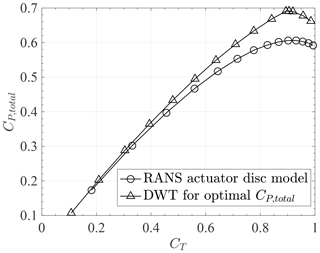Figure 9Comparison of CP,total vs. CT for RANS actuator disc model and the DWT for optimal CP,total.

## 3.1 Reynolds number sensitivity

To examine Reynolds number sensitivity, the simulation of the optimal design was repeated at $R{e}_{\mathrm{c}}=\mathrm{1.2}×{\mathrm{10}}^{\mathrm{6}}$. The Reynolds numbers used in this study correspond to typical values for DWTs, which typically target residential applications. For example, at a wind speed of V=10 m s−1 at standard sea-level temperature and pressure, the $R{e}_{\mathrm{c}}=\mathrm{3}×{\mathrm{10}}^{\mathrm{5}}$ design corresponds to c=0.44 m, D=2.4 m, and P=2.3 kW, and $R{e}_{\mathrm{c}}=\mathrm{1.2}×{\mathrm{10}}^{\mathrm{6}}$ corresponds to c=1.76 m, D=9.8 m, and P=38 kW.

The result of optimization at this higher Reynolds number is shown in the second row of Table 2. The maximum CP,total slightly increased to 0.70. The optimal value of $c/D$ did not change to the precision of the optimization. The optimal values of $\mathrm{\Delta }n/c$ and ${z}_{\mathrm{rotor}}/l$ also did not change, and the optimal CT and α only varied slightly. This confirms that at $R{e}_{\mathrm{c}}=\mathrm{3}×{\mathrm{10}}^{\mathrm{5}}$ the Reynolds number dependency was small.

Table 2The Reynolds number sensitivity of optimal design for CP,total.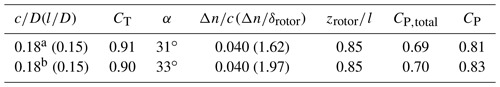a The optimization performed with $c/D$ as a design variable at $R{e}_{\mathrm{c}}=\mathrm{3}×{\mathrm{10}}^{\mathrm{5}}$.
b The optimization performed with $c/D$ as a design variable at $R{e}_{\mathrm{c}}=\mathrm{1.2}×{\mathrm{10}}^{\mathrm{6}}$.

The contour plot of eddy viscosity ratio $\frac{{\mathit{\mu }}_{t}}{\mathit{\mu }}$ for the optimal design is shown in Fig. 10a. On the suction side of the duct at $R{e}_{\mathrm{c}}=\mathrm{3}×{\mathrm{10}}^{\mathrm{5}}$ the turbulence model is activated near the leading edge of the duct. This suggests that the optimal design should be insensitive to Reynolds number. The eddy viscosity ratio at $R{e}_{\mathrm{c}}=\mathrm{1.2}×{\mathrm{10}}^{\mathrm{6}}$ is shown in Fig. 10b. At this Reynolds number the turbulence model is activated slightly further upstream and the power output increased to CP=0.82. The small increase can be explained by the reduced flow separation near the trailing edge at this greater Reynolds number, which increased the effective exit area of the duct. Also, note that the turbulence model on the pressure side becomes activated further upstream as well. However, this should not matter in identifying the optimal design as the flow stays attached on the outside of the duct. The kω SST turbulence model, with the apparent transition near the leading edge, indicates that the flow is entirely turbulent over the airfoil, and therefore the results should not change significantly even at higher Reynolds numbers.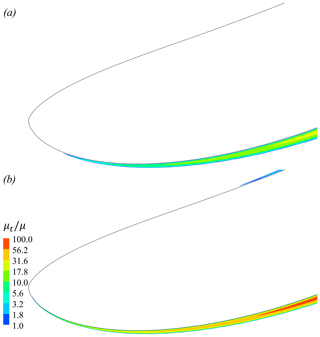Figure 10Eddy viscosity ratio contours near the leading edge of the duct for the optimal design ($c/D=\mathrm{0.18}$, CT=0.91, α=31, $\mathrm{\Delta }n/c=\mathrm{0.040}$, ${z}_{\mathrm{rotor}}/l=\mathrm{0.85}$) at (a) $R{e}_{\mathrm{c}}=\mathrm{3}×{\mathrm{10}}^{\mathrm{5}}$ and (b) $R{e}_{\mathrm{c}}=\mathrm{1.2}×{\mathrm{10}}^{\mathrm{6}}$.

4 Conclusions

The optimal design of a ducted wind turbine with the Eppler E423 airfoil as its cross section was investigated using CFD simulations of axisymmetric RANS equations with the kω SST turbulence model and an actuator disc. The total power coefficient characterizing the power output per total area of the device (CP,total) was used as the design objective. The design variables included the chord length and the angle of attack of the duct cross section, the thrust coefficient, the rotor axial position, and the tip clearance of the rotor.

The results demonstrate the existence of a maximum CP,total for ducted wind turbines, which sets an upper limit for ducted wind turbines similar to the Betz–Joukowsky limit for open rotors. For the Eppler E423 airfoil, this maximum was obtained for a duct length of about 15 % of the rotor diameter. With this duct length a CP,total of 0.70 was obtained, which exceeds what can be obtained with an open rotor by 16 %. This is the first time that an optimal duct length has been identified, although the optimization was for CP,total and not CP. The value of CP increased almost linearly with duct length over the range investigated.

Additionally, the results of optimization at fixed $c/D$ suggested that the optimal value of the design variables can significantly change with the duct length. In agreement with previous theoretical and numerical studies, for all duct lengths the optimal thrust coefficient (CT) was almost 0.9, which is similar to open rotor turbines. The results also showed that the optimal design for CP,total was most sensitive to the thrust coefficient of the rotor, which indicates the importance of proper rotor design. At lower-than-optimal thrust coefficients, the power per unit device area (CP,total) of the optimal DWT design gradually approached that of an open wind turbine. This can be explained by considering that the optimal design was on the verge of flow separation and the rotor became less effective in keeping the flow attached as the thrust coefficient decreased.

The optimal angle of attack of the duct cross section decreased significantly with increasing the duct length. Additionally, the optimal design was on the verge of flow separation with respect to the angle of attack of the duct cross section and the axial position of the rotor.

The optimal normal rotor gap was close to the boundary layer thickness at the rotor. Therefore, the optimal normal rotor gap scaled proportional to the chord length of the duct cross section as the turbulent boundary layer thickness almost linearly increases with the chord length of the duct cross section. This gap is needed to create the high-velocity annular jet, which helps keep the boundary layer attached.

The optimal rotor position was at the rear of the duct, but at greater values of duct length it moved further upstream in the duct. This further upstream position was more effective at eliminating flow separation and hence allowed greater values of CP,total.

Data availability

Data are available upon request from the corresponding author.

Author contributions

NBS contributed to the methodology, ran the simulations, post-processed the data, and wrote the first draft of the paper. BTH supervised the study and contributed to the conceptualization, methodology, writing, and revision of the paper. KDV contributed to the conceptualization and revision of the paper.

Competing interests

The authors declare that there is no conflict of interest.

Disclaimer

Publisher's note: Copernicus Publications remains neutral with regard to jurisdictional claims in published maps and institutional affiliations.

Review statement

This paper was edited by Jens Nørkær Sørensen and reviewed by Peter Jamieson, David Wood, and one anonymous referee.

References

Agha, A. and Chaudhry, H. N.: State-of-the-art in development of diffuser augmented wind turbines (DAWT) for sustainable buildings, MATEC Web Conf., 120, 08008, https://doi.org/10.1051/matecconf/201712008008, 2017. a

Aranake, A. and Duraisamy, K.: Aerodynamic optimization of shrouded wind turbines, Wind Energy, 20, 877–889, https://doi.org/10.1002/we.2068, 2017. a, b, c, d, e, f, g

Bagheri-Sadeghi, N., Helenbrook, B. T., and Visser, K. D.: Ducted wind turbine optimization and sensitivity to rotor position, Wind Energ. Sci., 3, 221–229, https://doi.org/10.5194/wes-3-221-2018, 2018. a, b, c, d, e, f, g, h, i, j, k, l, m, n, o, p, q, r, s, t, u, v, w

Bagheri-Sadeghi, N., Helenbrook, B. T., and Visser, K. D.: Wake comparison of open and ducted wind turbines using actuator disc simulations, in: Proceedings of the ASME 2020 Fluids Engineering Division Summer Meeting, 13–15 July 2020, Online, 3, V003T05A050, https://doi.org/10.1115/FEDSM2020-20300, 2020. a

Bontempo, R. and Manna, M.: Diffuser augmented wind turbines: Review and assessment of theoretical models, Appl. Energy, 280, 115867, https://doi.org/10.1016/j.apenergy.2020.115867, 2020. a, b

de Vries, O.: Fluid dynamic aspects of wind energy conversion, AGARDograph, AGARD, London, UK, 3-1–3-15, 1979. a

Foreman, K., Gilbert, B., and Oman, R.: Diffuser augmentation of wind turbines, Solar Energy, 20, 305–311, https://doi.org/10.1016/0038-092X(78)90122-6, 1978. a

Georgalas, C. G., Koras, A. D., and Raptis, S. N.: Parametrization of the power enhancement calculated for ducted rotors with large tip clearance, Wind Eng., 15, 128–136, 1991. a, b, c, d, e

Gilbert, B. L. and Foreman, K. M.: Experiments With a diffuser-augmented model wind turbine, J. Energ. Resour. Technol., 105, 46–53, https://doi.org/10.1115/1.3230875, 1983. a, b, c

Glauert, H.: Airplane Propellers, in: Aerodynamic Theory, vol. IV, edited by: Durand, W., Springer, Berlin, Germany, 169–360, 1935. a

Hansen, M. O. L., Sørensen, N. N., and Flay, R. G. J.: Effect of placing a diffuser around a wind turbine, Wind Energy, 3, 207–213, https://doi.org/10.1002/we.37, 2000. a, b, c, d

Hjort, S. and Larsen, H.: A multi-element diffuser augmented wind turbine, Energies, 7, 3256–3281, https://doi.org/10.3390/en7053256, 2014. a

Hooke, R. and Jeeves, T. A.: “ Direct search” solution of numerical and statistical problems, J. ACM, 8, 212–229, https://doi.org/10.1145/321062.321069, 1961. a

Igra, O.: Research and development for shrouded wind turbines, Energ. Convers. Manage., 21, 13–48, https://doi.org/10.1016/0196-8904(81)90005-4, 1981. a, b, c

Ishugah, T., Li, Y., Wang, R., and Kiplagat, J.: Advances in wind energy resource exploitation in urban environment: A review, Renew. Sustain. Energ. Rev., 37, 613–626, https://doi.org/10.1016/j.rser.2014.05.053, 2014. a

Jamieson, P.: Generalized limits for energy extraction in a linear constant velocity flow field, Wind Energy, 11, 445–457, https://doi.org/10.1002/we.268, 2008. a, b

Jones, A., Bakhtian, N., and Babinsky, H.: Low Reynolds number aerodynamics of leading-edge flaps, J. Aircraft, 45, 342–345, https://doi.org/10.2514/1.33001, 2008. a

Kanya, B. and Visser, K. D.: Experimental validation of a ducted wind turbine design strategy, Wind Energ. Sci., 3, 919–928, https://doi.org/10.5194/wes-3-919-2018, 2018. a

Kelley, C. T.: Iterative Methods for Optimization, Society for Industrial and Applied Mathematics, Philadelphia, USA, https://doi.org/10.1137/1.9781611970920, 1999. a

Kolda, T. G., Lewis, R. M., and Torczon, V.: Optimization by direct search: new perspectives on some classical and modern methods, SIAM Rev., 45, 385–482, https://doi.org/10.1137/S003614450242889, 2003. a

Lilley, G. and Rainbird, W.: A preliminary report on the design and performance of ducted windmills, Tech. rep., College of Aeronautics, Cranfield, 1956. a

Limacher, E. J., da Silva, P. O., Barbosa, P. E., and Vaz, J. R.: Large exit flanges in diffuser-augmented turbines lead to sub-optimal performance, J. Wind Eng. Indust. Aerodynam., 204, 104228, https://doi.org/10.1016/j.jweia.2020.104228, 2020. a

Loeffler Jr., A. L.: Flow Field Analysis and Performance of Wind Turbines Employing Slotted Diffusers, J. Solar Energ. Eng., 103, 17–22, https://doi.org/10.1115/1.3266198, 1981.  a, b, c

McLaren-Gow, S.: Rethinking Ducted Turbines: The Fundamentals of Aerodynamic Performance and Theory, PhD thesis, University of Strathclyde, Strathclyde, 2020. a

Menter, F. R.: Two-equation eddy-viscosity turbulence models for engineering applications, AIAA J., 32, 1598–1605, https://doi.org/10.2514/3.12149, 1994. a, b, c

Mikkelsen, R.: Actuator Disc Methods Applied to Wind Turbines, PhD thesis, Technical University of Denmark, Lyngby, Denmark, 2004. a

Ohya, Y. and Karasudani, T.: A shrouded wind turbine generating high output power with wind-lens technology, Energies, 3, 634–649, https://doi.org/10.3390/en3040634, 2010. a, b

Okulov, V. L. and van Kuik, G. A.: The Betz–Joukowsky limit: on the contribution to rotor aerodynamics by the British, German and Russian scientific schools, Wind Energy, 15, 335–344, https://doi.org/10.1002/we.464, 2012. a

Phillips, D. G., Richards, P. J., and Flay, R. G. J.: CFD modelling and the development of the diffuser augmented wind turbine, Wind Struct., 5, 267–276, 2002. a, b, c, d

Politis, G. K. and Koras, A. D.: A performance prediction method for ducted medium loaded horizontal axis windturbines, Wind Eng., 19, 273–288, 1995. a, b, c, d, e, f

Sanuki, M.: Studies on biplane wind vanes, ventilator tabes and cup anemometers (II), Pap. Meteorol. Geophys., 1, 227–298, 1950. a

Selig, M. S., Guglielmo, J. J., Broeren, A. P., and Giguere, P.: Summary of Low-Speed Airfoil Data – Vol. 2, SoarTech Publications, Virginia Beach, VA, 1996. a

Sørensen, J. N., Shen, W. Z., and Munduate, X.: Analysis of wake states by a full-field actuator disc model, Wind Energy, 1, 73–88, https://doi.org/10.1002/(SICI)1099-1824(199812)1:2<73::AID-WE12>3.0.CO;2-L, 1998. a, b

van Bussel, G. J. W.: The science of making more torque from wind: diffuser experiments and theory revisited, J. Phys.: Conf. Ser., 75, 012010, https://doi.org/10.1088/1742-6596/75/1/012010, 2007. a, b, c, d

Venters, R., Helenbrook, B. T., and Visser, K. D.: Ducted wind turbine optimization, J. Solar Energ. Eng., 140, 011005, https://doi.org/10.1115/1.4037741, 2018. a, b, c, d, e, f, g, h, i

White, F. M.: Viscous Fluid Flow, 3rd Edn., McGraw-Hill, New York, USA, 2006. a